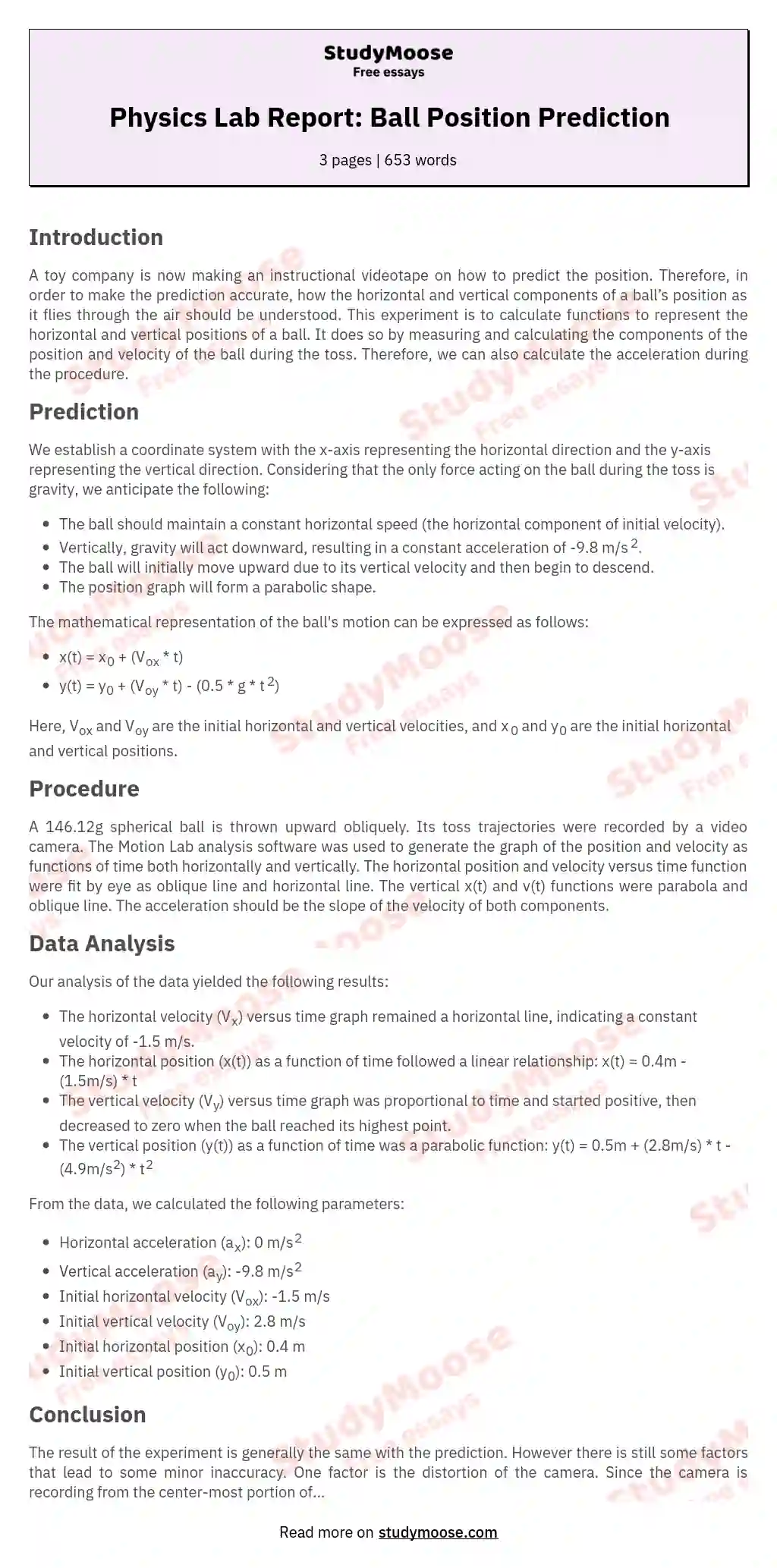# Physics lab report-motion

Introduction
A toy company is now making an instructional videotape on how to predict the position. Therefore, in order to make the prediction accurate, how the horizontal and vertical components of a ball’s position as it flies through the air should be understood. This experiment is to calculate functions to represent the horizontal and vertical positions of a ball. It does so by measuring and calculating the components of the position and velocity of the ball during the toss. Therefore, we can also calculate the acceleration during the procedure.

Prediction
The x-axis is located on the ground level horizontally, pointing to where the ball is initially thrown, that is opposite the direction the ball flies. The vertical y-axis passes through the highest point of the ball during the fly and point upward. Since the ball experiences no other force, except for gravity, during the toss. There is no horizontal force. It is predicted that the ball should have a constant horizontal speed, which is the horizontal component of initial velocity.

Get quality help nowWriterBelleVerified writer

Proficient in: Mechanical Engineering4.7 (657)

“ Really polite, and a great writer! Task done as described and better, responded to all my questions promptly too! ”+84 relevant experts are online

Vertically, it has gravity pulling it down all the time. So it should have an acceleration of –g (minus is for the direction). Since it has a vertical velocity, the ball should go up for a while and then fall down. The position graph should be a parabola. The function should be as follows:

The Vox and Voy are for the initial horizontal and vertical velocities and the x0 and y0 are for the initial horizontal the vertical position.

Procedure
A 146.12g spherical ball is thrown upward obliquely. Its toss trajectories were recorded by a video camera. The Motion Lab analysis software was used to generate the graph of the position and velocity as functions of time both horizontally and vertically. The horizontal position and velocity versus time function were fit by eye as oblique line and horizontal line. The vertical x(t) and v(t) functions were parabola and oblique line. The acceleration should be the slope of the velocity of both components.

Data Analysis
The horizontal velocity versus time graph is a horizontal line, which means
the velocity doesn’t change during the entire process, as is in the graph of position as a function of time, a straight line. Vx= – 1.5m/s

Xx=(0.4m)- (1.5m/s)t
The speed is constant, so the acceleration is 0m/s2. and the initial velocity is -1.5m/s. The initial position is 0.4m/

The vertical velocity is proportional to the time. Since it has an initial velocity. The position increases at the beginning and begins dropping when the velocity reaches 0. Vy=(2.8m/s)- (9.8m/s2)t

Xy=(0.5m)+ (2.8m/s)t- (4.9m/s2)t2
The acceleration is due to the gravity. The gravitational acceleration is 9.8m/s2. The initial velocity is 2.8 m/s, and the initial position is 0.5m.

The launch velocity of the ball is (-1.5, -3.0) while the velocity of the ball at the highest point is (-1.5, -0.2). However, the vertical velocity at the highest point should be 0m/s. But because of some experimental error, the value is not so accurate. And the acceleration of the ball is the derivative of the velocity. So the acceleration is (0, -9.8) throughout the entire process and is pointing downwards. Conclusion

The result of the experiment is generally the same with the prediction. However there is still some factors that lead to some minor inaccuracy. One factor is the distortion of the camera. Since the camera is recording from the center-most portion of the field, the graph it is plotting may not be so accurate. Another one is the air resistance. During the toss, the ball experience the air resistance to some extent both vertically and horizontally. However when we are doing the prediction, the resistance is neglected, But it does exist, though minor, in the actual experiment. In the procedure, we get -0.2m/s as the vertical velocity at the highest point. However as we predicted, it should be 0m/s. This is probably because the point we are choosing is not actually the real highest point. The camera is not precise enough to record any single change of the ball.

Generally the horizontal velocity does not change and the position is promotional to time. But the vertical velocity decreases and the vertical position increases and then decreases.The ball have the maxim velocity when it hit the ground and have the smallest at the highest point.